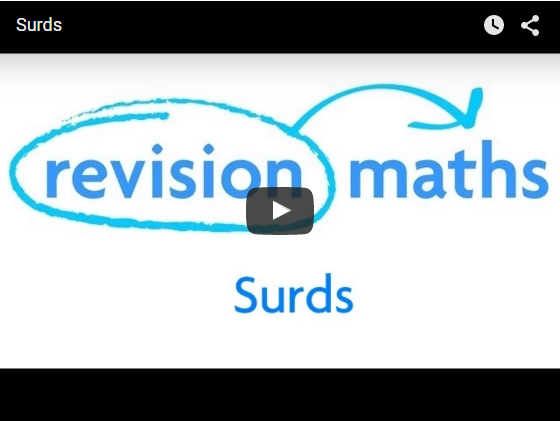## Surds

Surds are numbers left in 'square root form' (or 'cube root form' etc). They are therefore irrational numbers. The reason we leave them as surds is because in decimal form they would go on forever and so this is a very clumsy way of writing them.

The video below explains that surds are the roots of numbers that are not whole numbers. An example shows why surds are not written out as decimals because they are infinite decimals. Rules of working with surds are outlined and it is demonstrated how they can be simplified and rationalised.Multiplication of Surds

√5 × √15 = √75 (= 15× 5)

= √25 × √3

= 5 √3

(1 + √3)(2 - √8) [The brackets are expanded as usual]

= 2 - √8 + 2√3 - √24

= 2 - 2√2 + 2√3 - 2√6

Adding and subtracting surds are simple- however we need the numbers being square rooted (or cube rooted etc) to be the same.

4√7 - 2√7 = 2√7.

5√2 + 8√2 = 13√2

Note: 5√2 + 3√3 cannot be manipulated because the surds are different (one is √2 and one is √3).

However, if the number in the square root sign isn't prime, we might be able to split it up in order to simplify an expression.

Example

Simplify √12 + √27

12 = 3 × 4. So √12 = √(3 × 4) = √3 × √4 = 2 × √3.

Similarly, √27 = 3√3.

Hence √12 + √27 = 2√3 + 3√3 = 5√3

Rationalising the Denominator (HIGHER TIER)

It is untidy to have a fraction which has a surd denominator. This can be 'tidied up' by multiplying the top and bottom of the fraction by a particular expression. This is known as rationalising the denominator, since surds are irrational numbers and so you are changing the denominator from an irrational to a rational number.

Example

Rationalise the denominator of:

a) 1

√2

b) 1 + 2

1 - √2

a) Multiply the top and bottom of the fraction by √2. The top will become √2 and the bottom will become 2 (√2 times √2 = 2).

b) In situations like this, look at the bottom of the fraction (the denominator) and change the sign (in this case change the minus into a plus). Now multiply the top and bottom of the fraction by this.

Therefore:

1 + 2 = (1 + 2)(1 + √2) = 1 + √2 + 2 + 2√2 = 3 + 3√2

1 - √2   (1 - √2)(1 + √2)   1 + √2 - √2 - 2          - 1

= -3(1 + √2)

You can find out more about surds by clicking here